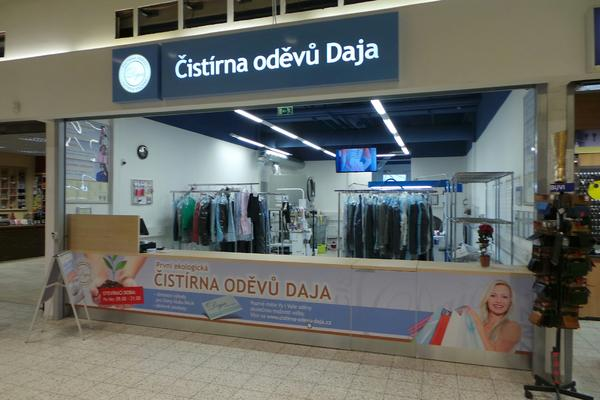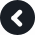Accept
This page uses cookies. Continuing using this web you agree with this.

# Dry Cleaning Prague 5

Address: HM Globus Zličín, Sárská 5, Praha 5

Telephone: +420 235 316 826

Opening hours:
Mon - Sun 8.00 am – 8.00 pm

How to get to us:
Metro station: B - Zličín
Autobus: Zličín
Parking available on sitex x x x x x x x x x x x x x x x x x x x x x x x x x x x x x x x x x x x x x x x x x x x x x x x x x x x x x x x x x x x x x x x x x x x x x x x x x x x x x x x x x x x x x x x

## Our Awards